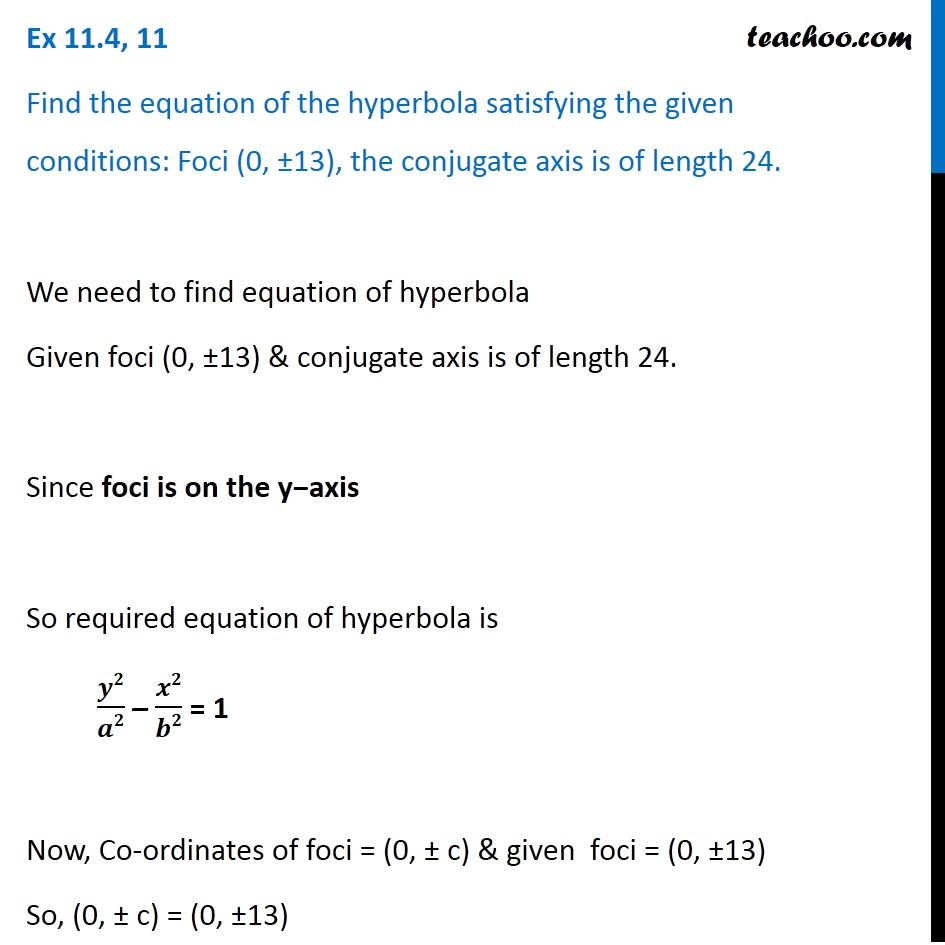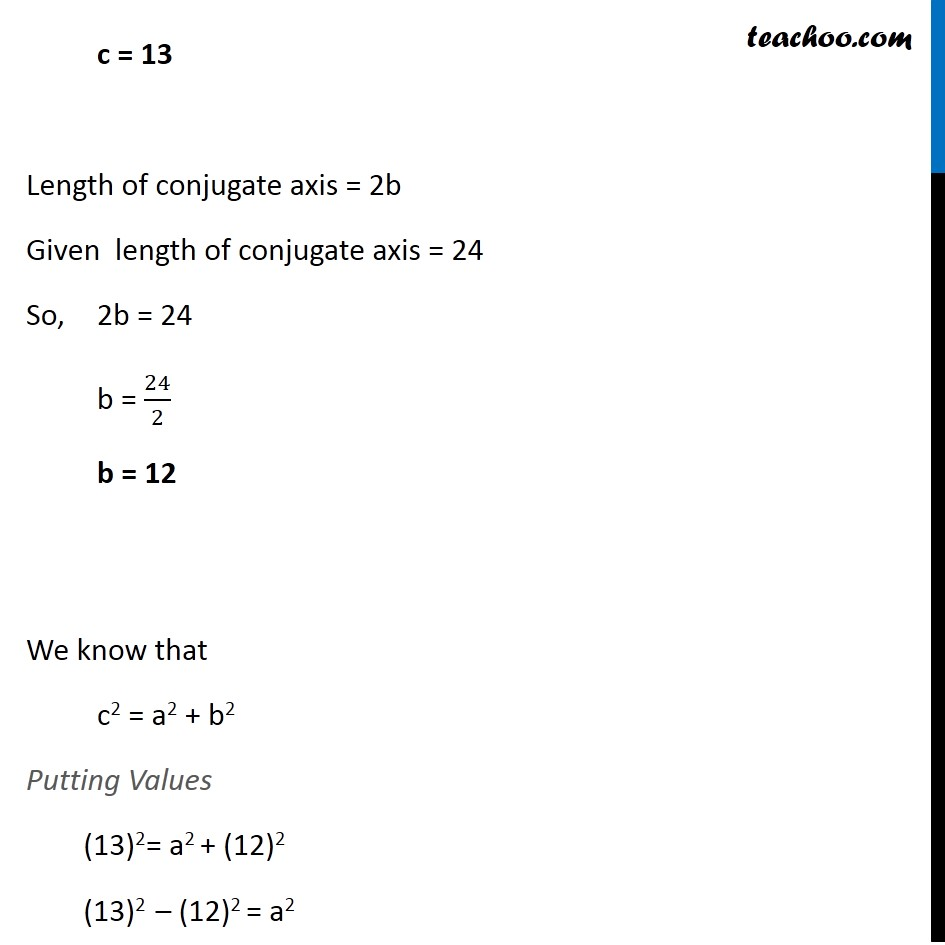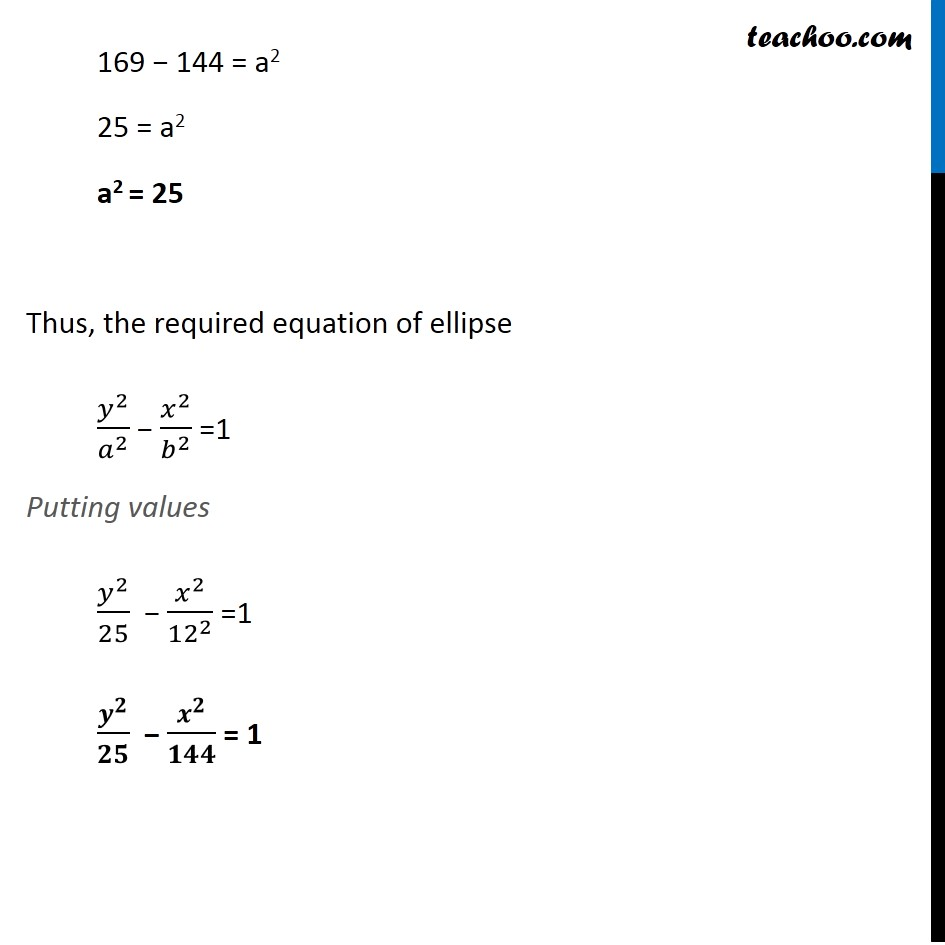Ex 10.4

Chapter 10 Class 11 Conic Sections
Serial order wiseLearn in your speed, with individual attention - Teachoo Maths 1-on-1 Class

### Transcript

Ex 10.4, 11 Find the equation of the hyperbola satisfying the given conditions: Foci (0, ±13), the conjugate axis is of length 24. We need to find equation of hyperbola Given foci (0, ±13) & conjugate axis is of length 24. Since foci is on the y−axis So required equation of hyperbola is 𝒚𝟐/𝒂𝟐 – 𝒙𝟐/𝒃𝟐 = 1 Now, Co-ordinates of foci = (0, ± c) & given foci = (0, ±13) So, (0, ± c) = (0, ±13) c = 13 Length of conjugate axis = 2b Given length of conjugate axis = 24 So, 2b = 24 b = 24/2 b = 12 We know that c2 = a2 + b2 Putting Values (13)2= a2 + (12)2 (13)2 – (12)2 = a2 169 − 144 = a2 25 = a2 a2 = 25 Thus, the required equation of ellipse 𝑦^2/𝑎^2 − 𝑥^2/𝑏^2 =1 Putting values 𝑦^2/25 − 𝑥^2/〖12〗^2 =1 𝒚^𝟐/𝟐𝟓 − 𝒙^𝟐/𝟏𝟒𝟒 = 1